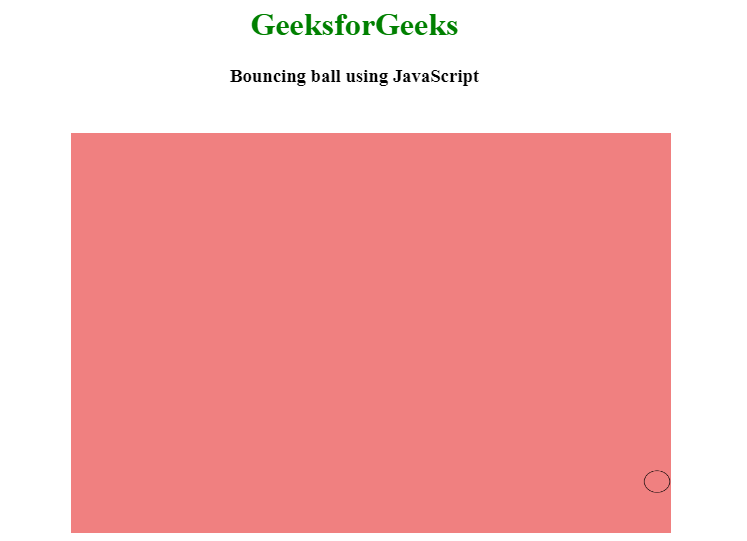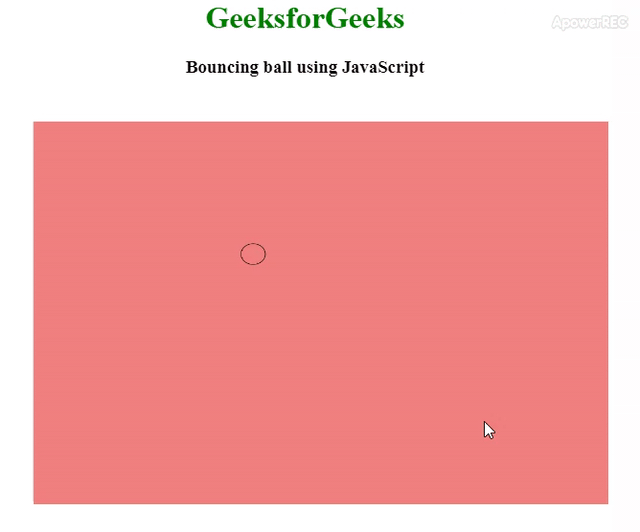Related Articles

# How to Build a Bounce Ball with HTML and JavaScript ?

• Difficulty Level : Basic
• Last Updated : 05 Jul, 2021Bouncing ball can be created by using HTML, CSS, and JavaScript and perform some bouncing operations on that ball. You can see related article how to make smooth bounce animation using CSS.
This article will be divided into two portions, 1st portion we will decide the area where the bouncing ball will perform bouncing, basically, we will create a canvas where bouncing will be performed. 2nd portion will design the bouncing ball and add some bouncing functionality on it.
HTML & CSS code: HTML and CSS code is used to create a canvas area where the ball will bounce. We will use a canvas tag and by using JavaScript we will struct the circle for the ball inside of that canvas. And the canvas area and background color of the canvas area is defined by the CSS.

## html

 ```<``html``>` `<``head``>``    ``<``title``>``        ``Bouncing Ball!!``    ````    ``<``style``>``        ``h1 {``            ``color: green;``        ``}``        ` `        ``canvas {``            ``background-color: #F08080;``            ``width: 600px;``            ``height: 400px;``            ``position: absolute;``            ``top: 20%;``            ``left: 20%;``        ``}``    ````` `<``body``>``    ``<``center``>``        ``<``h1``>GeeksforGeeks``        ``<``h3``>Bouncing ball using JavaScript``        ``<``canvas``>``        ````    ````` ``

JavaScript code: It is the core part of this article where we will struct the ball and perform the bouncing task. We will assign 4 variables, 2 for the created circle(ball) coordinates and others two for the respective speed of the bouncing ball. The radius variable is used for the ball’s radius. We also need to clear the canvas area to do so we will use the clearReact() function. All the bouncing and coordinate will decided by the math.random() function.

## javascript

 ``

Complete code: It is the combination of above two sections i.e. combining HTML, CSS and JavaScript code. This code will create an output where a design vall will bounce in random pattern.

## html

 ```<``html``>` `<``head``>``    ``<``title``>``        ``Bouncing Ball!!``    ````    ``<``style``>``        ``h1 {``            ``color: green;``        ``}``        ` `        ``canvas {``            ``background-color: #F08080;``            ``width: 600px;``            ``height: 400px;``            ``position: absolute;``            ``top: 20%;``            ``left: 20%;``        ``}``    ``` `` `<``body``>``    ``<``center``>``        ``<``h1``>GeeksforGeeks``        ``<``h3``>Bouncing ball using JavaScript``        ``<``canvas``>``        ````        ``<``script``>``            ``var canvas = document.querySelector("canvas");` `            ``canvas.width = window.innerWidth;``            ``canvas.height = window.innerHeight;` `            ``var l = canvas.getContext('2d');` `            ``// x and y are the co-ordinates of the circle` `            ``// vx and vy are the respective speeds` `            ``var x = Math.floor(Math.random() * innerWidth);``            ``var y = Math.floor(Math.random() * innerHeight);``            ``var vx = Math.floor(Math.random() * 2);``            ``var vy = Math.floor(Math.random() * 4);``            ``var radius = 20;` `            ``move();``          ` `            ``// This function will do the animation``            ``function move() {``                ``requestAnimationFrame(move);` `                ``// It clears the specified pixels within``                ``// the given rectangle``                ``l.clearRect(0, 0, innerWidth, innerHeight);` `                ``// Creating a circle``                ``l.beginPath();``                ``l.strokeStyle = "black";``                ``l.arc(x, y, radius, 0, Math.PI * 2, false);``                ``l.stroke();` `                ``// Conditions sso that the ball bounces``                ``// from the edges``                ``if (radius + x > innerWidth)``                    ``vx = 0 - vx;` `                ``if (x - radius < ``0``)``                    ``vx` `= ``0` `- vx;` `                ``if (y + radius > innerHeight)``                    ``vy = 0 - vy;` `                ``if (y - radius < ``0``)``                    ``vy` `= ``0` `- vy;` `                ``x` `= x + vx;``                ``y` `= y + vy;` `            ``}``        ````    ````` ``

Output:Attention reader! Don’t stop learning now. Get hold of all the important HTML concepts with the Web Design for Beginners | HTML  course.

My Personal Notes arrow_drop_up